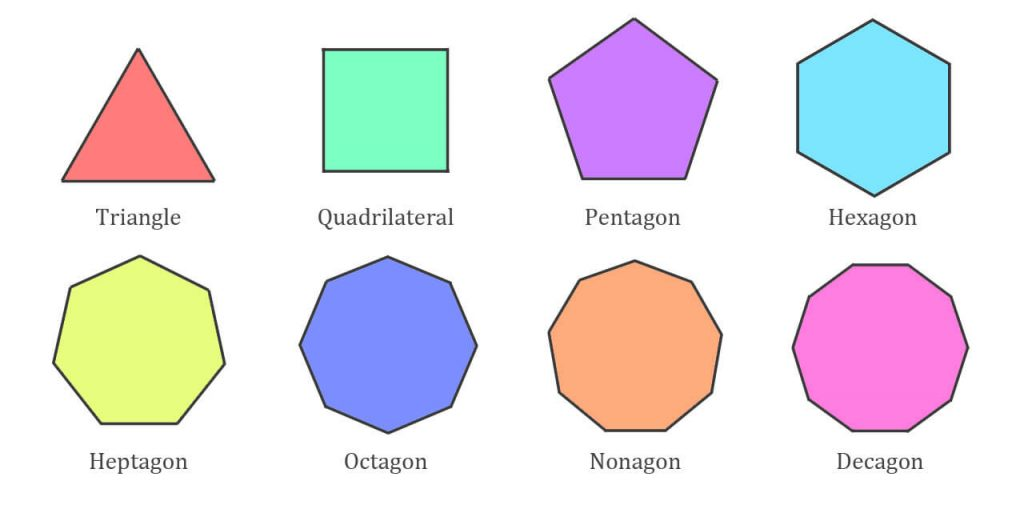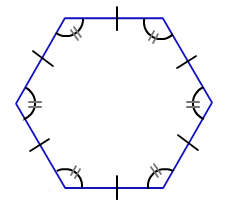Latest SSC jobs   »   Government Jobs   »   Polygon Definition, Types of Polygon, Formula...

# Polygon Definition, Types, Formula And Examples

## Polygon

Polygons are 2-dimensional shapes made up of straight lines and enclosed within sides. Polygon means all the closed shapes with straight-line figures come under the category of a polygon. You must know about the definition, shape, types, formula, and examples of a polygon by reading the article given below.

## Polygon Definition

A polygon is an enclosed shape with straight lines. 2-dimensional shapes like Rectangles, squares, etc are categorized under polygons. Polygons have a finite number of sides. A circle is not a polygon as it has a curved shape. The points where the 2 straight lines meet are called vertices. The interior is called the body.### Types of Polygon

There are mainly 2 types of Polygon:

1. Regular Polygon- The polygon which has equal sides and equal angles. Generally, questions from regular polygon are asked in the exam.
2. Irregular Polygon- The one with unequal sides and angles.

The below figures show Regular and Irregular Polygons:### Properties of Polygon

• Polygon: It is a closed plane figure bounded by three or more than three straight lines.
• Regular Polygon: All the sides are equal and also all the interior angles are equal

Sum of Interior angles of a polygon = (n – 2) × 180

n → number of sides

The sum of exterior angle = 360

Different Types Of Polygons
Name Sides Interior Angle
Triangle (or Trigon) 3 60°
Pentagon 5 108°
Hexagon 6 120°
Heptagon (or Septagon) 7 128.571°
Octagon 8 135°
Nonagon (or Enneagon) 9 140°
Decagon 10 144°
Hendecagon (or Undecagon) 11 147.273°
Dodecagon 12 150°
Triskaidecagon 13 152.308°
Tetrakaidecagon 14 154.286°
Hexakaidecagon 16 157.5°
Octakaidecagon 18 160°
Icosagon 20 162°
n-gon n (n-2) × 180° / n

### Important Formulae Related to Regular Polygon :

The important Polygon formulas have been listed below including the formula for the area of a polygon.## Question-based on polygons

Q1.The ratio between the number of sides of two regular polygons is 1: 2 and the ratio between their interior angles is 2 : 3. The number of sides of these polygons is respectively:
(a) 3, 6
(b) 5, 10
(c) 4, 8
(d) 6, 12Q2. If each interior angle of a regular polygon is 3 times its exterior angle, the number of sides of the polygon is :
(a) 4
(b) 5
(c) 6
(d) 8Q3. A polygon has 54 diagonals. The number of sides in the polygon is :
(a) 7
(b) 9
(c) 12
(d) 19Q4. The ratio between the number of sides of two regular polygon 1 : 2 and the ratio between their interior angle is 3 : 4. The number of sides of these polygons are respectively :
(a) 3, 6
(b) 4 , 8
(c) 6, 9
(d) 5, 10Q5. The sum of all the interior angles of a regular polygon is four times the sum of its exterior angles. The polygon is :
(a) hexagon
(b) triangle
(c) decagon
(d) nonagonQ6. The ratio of the measure of an angle of a regular nonagon to the measure of its exterior angle is :
(a) 3 : 5
(b) 5 : 2
(c) 7 : 2
(d) 4 : 5Q7.The ratio of the measure of an interior angle of a regular octagon to the measure of its exterior angle is :
(a) 1 : 3
(b) 2 : 3
(c) 3 : 1
(d) 3 : 2Q8.The sum of the interior angles of the polygon is 1440°. The number of sides of the polygon is :
(a) 9
(b) 10
(c) 8
(d) 12Q9.The sum of all exterior angles of a convex polygon of n sides is :
(a) 4 right angle
(b) 2/n right angle
(c) (2n – 4) right angle
(d) n/2 right angleQ10.One angle of a pentagon is 140°. If the remaining angles are in the ratio 1: 2 : 3: 4, the size of the greatest angle is :
(a) 150°
(b) 180°
(c) 160°
(d) 170°## FAQs

### What is polygon formula?

The important polygon formulas are The sum of interior angles of a polygon with “n” sides =180°(n-2) Number of diagonals of an “n-sided” polygon = [n(n-3)]/2

### What is polygon explain different types of polygon?

A Polygon is made up of only straight lines. Each straight line in a Polygon is called its side

#### Congratulations!General Awareness & Science Capsule PDF